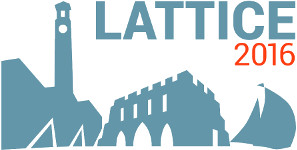#The 34th International Symposium on Lattice Field Theory (Lattice 2016)

Jul 24 – 30, 2016
Highfield Campus, University of Southampton
Europe/London timezone

## Glueball spectrum from $N_f=2$ lattice QCD study on anisotropic lattices

Jul 26, 2016, 5:50 PM
20m
Building B2a Room 2065 (Highfield Campus, University of Southampton)

### Speaker

Prof. Ying Chen (Institute of High Energy Physics, CAS)

### Description

The glueball spectrum is investigated through a $N_f=2$ lattice QCD study. The gauge configurations are generated with two degenerate flavors of quarks on anisotropic lattices. At two pion masses, say, $m_\pi=580$ MeV and $920$ MeV, we obtain the masses of the scalar, the tensor glueballs, which are in agreement with the results from the previous quenched and unquenched lattice QCD studies. For the pseudoscalar channel, we can get a state of mass roughly 2.4-2.5 GeV by the use of the gluonic lattice operators whose continuum counterparts are $\epsilon_{ijk}B_i^a(D_j B_k)^a$. This state is compatible with the pseudoscalar glueball from the previous quenched lattice QCD studies. We do not observe a clear quark mass dependence of the masses of these states. We also calculate the correlation functions of the topological charge density adopting the gradiant flow smearing scheme. We can seemingly observe a state with a mass around 1 GeV, but fail to obtain a definite result owing to our coarse and small lattices. This state might be the isoscalar $q\bar{q}$ pseudoscalar meson, the $SU(2)$ counterpart of the $SU(3)$ flavor singlet $\eta'$.

### Primary author

Prof. Ying Chen (Institute of High Energy Physics, CAS)

### Co-authors

Prof. Chuan Liu (Peking University) Prof. Jian-Bo Zhang (Zhejiang University) Prof. Jianping Ma (Institute of Theoretical Physics, CAS) Dr Long-Cheng Gui (Hunan Normal Unviersity) Dr Ming Gong (Institute of High Energy Physics, CAS) Mr Wei Sun (Institute of High Energy Physics, CAS) Prof. Yu-Bin Liu (Nankai University) Dr Zhaofeng Liu (Institute of High Energy Physics, CAS)

 Slides